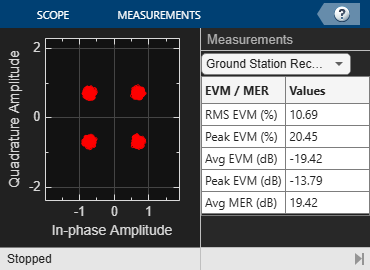# Interference Modeling

This example shows interference modeling in a bent pipe satellite communications link using Communications Toolbox™.

### Introduction

Signal interference is the addition of unwanted signals to a desired signal and is a common problem in many communications systems. Some examples of interference are:

1. The coexistence of 5G and LTE waveforms in the same or similar frequency bands results in one waveform interfering with another waveform

2. Signals from a secondary base station interfering with the signal from the primary base station at a mobile device

4. Interference occurs when a satellite receives and re-broadcasts a strong uplink signal from secondary ground station

Modeling such interference scenarios allows you to analyze their impact on system performance and to design migitation strategies.

### System Setup

This example models a bent pipe satellite communication link and illustrates how to model an uplink interference scenario. A bent pipe link consists of an uplink from a ground station to a satellite, which acts as a repeater, and downlinks to another ground station without performing any bit-level processing. The satellite transponder receives a primary signal and an interfering signal from a secondary ground station. The combined signal is re-broadcast by the satellite, received and processed at the ground station.

A Signal Aggregator block depicts an efficient approach to combine the primary and the interfering signals at baseband. The Signal Aggregator block is configured to interpolate the two signals so that the resulting sample rate of the signals guarantees no loss of spectral content when the signals are frequency shifted to model the interference scenario. Then it applies the specified frequency shifts to the signals and combines them into one signal. The block allows modeling of various amount of signal overlap to simulate varying severity of interference. The following block diagram illustrates the SignalAggregator processing:### System Simulation

Each of the two baseband signals has a bandwidth of 500 kHz as seen in the Tx Signal Spectrum scope. The 'Desired output center frequency' parameter of Signal Aggregator block is set up to model spectral overlap of 100 kHz. This spectral overlap is seen in the Rx Signal Spectrum that shows the spectra of the signals received at the satellite transponder and ground station receiver.

A bit error rate of 0 shows that the system performance is not degraded by this amount of interference. Also, the Received Signal Constellation at the ground station receiver is well clustered around the reference QPSK constellation of the primary signal with a low RMS EVM.

```modelName = 'InterferenceModelingExample'; open_system(modelName); sim(modelName); ```Increase the interference effect by increasing the spectral overlap between the two signals. The increased interference degrades the system performance, as seen from the nonzero bit error rate and a more spread out received signal constellation with higher RMS EVM.

```set_param([modelName '/Signal Aggregator'], 'CenterFrequency', '[0 360e3]'); sim(modelName); ``````close_system(modelName, 0); ```

### Summary and Further Exploration

This example illustrates a technique to model signal interference that is common in many wireless communications systems. A subsystem-based block shows the necessary processing of interpolation, frequency shift and signal combining required to simulate various interference scenarios. Other ways to explore interference with this model include:

1. Using baseband signals with different bandwidths

2. Activating and deactivating interference using the switch in 'Interfering Signal' subsystem

3. Modeling more than two baseband signals and more than one interfering signal

4. Modeling various amount of interference by setting parameters of Signal Aggregator block appropriately

5. Modeling various approaches to minimize the impact of interference at the satellite transponder and ground station receiver

Experiment with the Signal Aggregator block and possibly alter the processing necessary for the particular interference scenario. In 'Auto' Interpolation mode, the Signal Aggregator block interpolates the two signals such that the frequency content of the original signals is not distorted after they are frequency shifted. You can also interpolate the baseband input signals to the rate you desire before using Signal Aggregator block and use the 'None' Interpolation mode of the block which will not perform any interpolation. This example uses two signals, but the block can process any number of input signals once they are concatenated into a matrix.

'Multiband Signal Generation' example illustrates a SignalAggregator System object™ to perform similar processing as Signal Aggregator block in MATLAB®.